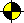Prolog Guide - Representing Data Structures

Guide to Prolog Programming

Home
First Steps in Prolog

### Representing Data StructuresThis lesson covers data structures in Prolog. The basic data structure in Prolog is term which is expressed in form name(arguments...). If the number of arguments is zero then we speak about atom. A special type of atom is number.

### Basics (Dates example)

In this section, we introduce a data structure date(Year,Month,Day) that represents date. First, we need a "constructor" of data structure date that makes the data structure from year, month and day:

`make_date(Y,M,D,date(Y,M,D)).`

Second, we define functions to access components of data structure in a following way:

```get_year(date(Y,_,_),Y).
get_month(date(_,M,_),M).
get_day(date(_,_,D),D).```

get_xxx can be used to test or generate correspondent component of data structure, but it can not be used to set the value of the component. So, we have to define set_xxx to set values of components of data structure date.

```set_year(Y,date(_,M,D),date(Y,M,D)).
set_month(M,date(Y,_,D),date(Y,M,D)).
set_day(D,date(Y,M,_),date(Y,M,D)).```

Now, it is easy to find the "same" day in next or previous year respectively using get and set functions.

```next_year(Today,NextYear):-
get_year(Today,Y), NY is Y+1, set_year(NY,Today,NextYear).
prev_year(Today,PrevYear):-
get_year(Today,Y), PY is Y-1, set_year(PY,Today,PrevYear).```

Note, that following definition of prev_year using next_year is not correct. Do you know why?

`prev_year(Today,PrevYear):-next_year(PrevYear,Today). % incorrect`

Finding next year is relatively easy but what about finding next day, i.e., tomorrow? Study following program to find where the possible problems are hidden. The definition of test correct_day follows the next section that covers working with lists.

```tomorrow(Today,Tomorrow):-
get_day(Today,D), ND is D+1, set_day(ND,Today,Tomorrow),
correct_date(Tomorrow).
% day inside month (i.e., not last day of month)
tomorrow(Today,Tomorrow):-
get_month(Today,M), NM is M+1,
set_month(NM,Today,Tmp), set_day(1,Tmp,Tomorrow),
correct_date(Tomorrow).
% last day of month
tomorrow(Today,Tomorrow):-
get_year(Today,Y), NY is Y+1, make_date(NY,1,1,Tomorrow).
% last day of year```

Note that it is also possible and probably more reasonable to encapsulate the test correct_date to definitions of make_date and set_xxx.

### Lists

List is a widely used data structure which is build in Prolog. It is still a term, e.g., [1,2,3] is equivalent to '.'(1,'.'(2,'.'(3,nil))). The following functions enable access to list elements.

```head(H,[H|_]).
tail(T,[_|T]). % T is list```

It is easy to access the first element of list as it corresponds to the head. However, finding the last element is a time consuming process as one has to go through the whole list to find it. Note that following "procedures" can be used to find the first/last element of list as well as to test whether given element is first/last element of list. It could even be used to generate list with given first/last element.

```first(F,[F|_]). % the same as head
last(L,[L]).
last(L,[H|T]):-last(L,T).```

The similar conclusion also holds for finding prefix and suffix respectively. Again, the same procedure can be used to test or generate prefix/suffix respectively as well as to generate list with given prefix/suffix. Try it.

```prefix([],_).
prefix([H|T1],[H|T2]):-prefix(T1,T2).
suffix(S,S).
suffix([H|T],L):-suffix(T,L).```

Testing membership is an important method for working with lists. Prolog definiton of member can test membership relation as well as generate successive members of list. A similar function, nth_member, can also be used to test or to generate n-th member of list. However, it can not be used to count a sequence number of given element (define the function that counts a sequence number of given element as your homework).

```member(X,[X|_]).
member(X,[_|T]):-member(X,T).
nth_member(1,[M|_],M).
nth_member(N,[_|T],M):-N>1, N1 is N-1, nth_member(N1,T,M).```

Another popular function on lists is append which appends a list to another list. It can be also used to disjoint list (see following definition of prefix and suffix).

```append([],L,L).
append([H|T],L,[H|LT]):-append(T,L,LT).```

Now, prefix and suffix relations can be easily redefined using append:

```prefix(P,L):-append(P,_,L).
suffix(S,L):-append(_,S,L).```

append can be successfuly used in many other operations with lists including testing (or generating) sublist. The following rule exploits again the declarative character of Prolog.

`sublist(S,L):-append(_,S,P),append(P,_,L).`

There are (at least) two other ways how to define sublist, e.g., using prefix and suffix relations. All these definitions are equivalent. However, the procedure sublist3 is probably the closest to traditional (non-declarative) programming style as it uses technique known as "floating window".

```sublist2(S,L):-prefix(P,L),suffix(S,P).

sublist3(S,L):-prefix(S,L).
sublist3(S,[_|T]):-sublist3(S,T).```

### Back to Dates example

Let us return to our example of data structure date. Now, we can define the test correct_date using lists.

First, we add two facts to Prolog database with distribution of days:

```year(regular,[31,28,31,30,31,30,31,31,30,31,30,31]).
year(leap,[31,29,31,30,31,30,31,31,30,31,30,31]).```

Then, we prepare the test of leap years (simplified version):

```year_type(Y,leap):-
Z is Y mod 4, Z=0. % every fourth year is leap (simplied)
year_type(Y,regular):-
Z is Y mod 4, Z\=0.```

Finally, it is possible to test correctness of date:

```correct_date(date(Y,M,D)):-
correct_month(M),
correct_day(Y,M,D).
correct_month(M):- M>0, M<13.
correct_day(Y,M,D):-
year_type(Y,Type),
test_day_of_year(Type,M,D).
test_day_of_year(Type,M,D):-
year(Type,Days),
nth_member(M,Days,Max),
D>0, D=<Max.```

Choice of appropriate data structure can simplify the programming of algorithm.